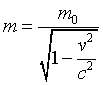QUESTION #180

# My science data book says that the mass of a photon is zero. But how can photon exert radiation pressure, as it occurs in the sun?

Asked by: Suraj

### Answer

Actually what your science book says or is trying to say anyway,is that the photon's rest mass is zero. The concept of the rest mass derives from special relativity. The rest mass is the mass of a particle (in our case the photon) as measured by an observer who sees the particle still and with zero speed. In other words, the particle is at rest as far as this observer is concerned. Thus comes the term REST mass. But according to special relativity, light ALWAYS travels with the light speed c, and is NEVER at rest. And so it has zero REST mass.

The mass of a particle which travels with a velocity v is given by the following equation:where m0 is the rest mass of the particle, v its velocity and c the speed of light. It may appear that because photons have zero rest mass (m0=0), their mass is zero too. A closer look at the equation will show that this is not the case because photons travel with the velocity c and the equation collapses to an undefined form (m=0/0) The conclusion is that this equation applies only to sub-lightspeed particles and NOT to photons. But relativity also tells us that every particle with mass m is equal to an energy E given by the famous equation:

E=mc2

where m the mass of the particle, c the speed of light and E the energy that equals to the mass m. This equation also works backwards.An amount of energy E equals to a mass m. Photons however may not have rest mass, but they DO have energy. The energy of a photon is given by the equation:

E=hf

where h is a constant (Planck's constant) and f the frequency of the photon (don't forget that the photon is an electromagnetic WAVE and has a frequency). If we combine these two equations, we come up with the following,which gives us the mass of a photon

mc2 = hf => m=hf/c2

which means that though photons don't have rest mass, they do have energy and thus they have mass. The photons are wave particles. This means that they act as waves and as particles as well. This is the duality of the nature of light (and of every particles). And so as particles they have mass, and as waves they have frequency. The pressure they exert is due to the particle nature of light. It's easy now to understand the mechanism that causes this pressure.
Answered by: George Moustris, M.S., Electrical and Computer Engineer, AUTH, Greece

Though the mass of a photon is zero, it nevertheless carries energy and momentum. The two are related through

p = E/c

where c is the speed of light and p is the momentum and E the energy of the photon.

When a photon strikes a surface, it may be absorbed or reflected. In either case, momentum is transferred from the photon to the object whose surface is struck. In this way, a force (time rate of change of momentum) is exerted on the struck object, giving rise to the notion of 'radiation pressure.'

If the photon is absorbed, the struck object acquires the momentum of the photon. If the photon is reflected, so that the photon rebounds with the same magnitude of momentum, but oppositely directed, conservation of momentum demands that the momentum transferred to the struck object is twice the (magnitude of the) momentum of the incoming photon.

Generally, if a beam of photons strikes a surface, some photons will be reflected and some will be absorbed. Therefore, the radiation pressure exerted by a beam of light incident upon a surface falls somewhere in the range between the minimum theoretical value when all incident photons are absorbed and the maximum theoretical value when all incident photons are reflected.
Answered by: Warren F. Davis, Ph.D. Physics, President, Davis Associates, Inc., Newton, MA USA